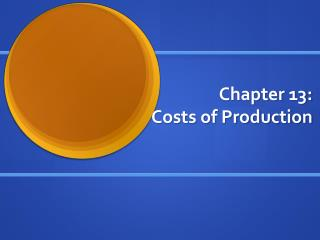Download PresentationChapter 13: Costs of Production

# Chapter 13: Costs of Production - PowerPoint PPT Presentation

Chapter 13: Costs of Production. The Supply and Demand . In Economy, Supply and Demand Basically runs all market activity. Supply and Demand is the most basic and the most important prefecture of Economy. Law of Supply.I am the owner, or an agent authorized to act on behalf of the owner, of the copyrighted work described.
Download Presentation## Chapter 13: Costs of Production

An Image/Link below is provided (as is) to download presentation

Download Policy: Content on the Website is provided to you AS IS for your information and personal use and may not be sold / licensed / shared on other websites without getting consent from its author.While downloading, if for some reason you are not able to download a presentation, the publisher may have deleted the file from their server.

- - - - - - - - - - - - - - - - - - - - - - - - - - E N D - - - - - - - - - - - - - - - - - - - - - - - - - -
Presentation Transcript
1. Chapter 13: Costs of Production

2. The Supply and Demand • In Economy, Supply and Demand Basically runs all market activity. • Supply and Demand is the most basic and the most important prefecture of Economy

3. Law of Supply • In the Law of Supply implies that a firm is willing to produce quantity of good, if the price is high enough • Causing Supply curve to go upwards.

4. Revenue, Cost, and Profit • Firms always try to maximize their revenue, and profit. • Total Revenue: The amount received after selling the product. • Total Cost: How much it costs to create a single item. • Total Revenue – Total Cost = Profit

5. Firm’s Profits • In the Firm’s Cost, there exists the Explicit Cost, and the Implicit Cost • Explicit Cost: Direct outlay of money • Implicit Cost: Cost not exactly requiring tangible money

6. Economic Profit vs. Accounting Profit • Economist view profit with total revenue minus Explicit cost Minus Implicit Cost. • Accountants view profit with total revenue minus only the Explicit Cost • Therefore making the Economic Profit less than Accounting Profit

7. The Production • In production, there are Key Terms to be realized. • Such as Marginal Product: any increase in the input process, for additional units • Diminishing Marginal Product: Rule which says, Marginal quantity decreases as Q of input increases

8. Costs of Production • Fixed Costs: Costs that do not change with the amount of input or output used. • Variable Cost: Costs that change with the amount of item a firm produces. • Total Cost: • Total Cost = Total Fixed Cost + Total Variable Cost

9. Cost of Production • Average Cost: Average cost is determined by the amount cost divided by the amount produced. • Average Total Cost = Average Fixed Cost + Average Variable Cost

10. Marginal Cost • MC equals Amount of Cost, by the Amount of quantity

11. ATC • ATC is U Shaped if graphed • ATC declines as output increases • ATC starts rising because variable cost rises

12. ATC • At the Bottom of the ATC curve may result in minimizing ATC, which becomes the • EFFICIENT SCALE OF ECONOMY

13. MC and ATC • Whenever MC is less than ATC, ATC is falling • Whenever MC is greater than ATC, the ATC is rising.

14. Econmy and Diseconomist • Diseconomy of Scale: refer to the property whereby long-run average total cost rises as the quantity of output increases. • Constant Returns of Scale: The marginal-cost curve crosses the average-total-cost curve at the minimum of average total cost.